TitleCollege Algebra
Tutorial 54C: Arithmetic Sequences and
SeriesLearning Objectives

 After completing this tutorial, you should be able to: Know what an arithmetic sequence is. Find the nth term of an arithmetic sequence. Write the formula for the nth term of an arithmetic sequence. Calculate an arithmetic series.Introduction

 In this tutorial we will mainly be going over arithmetic sequences and series.  We will be going forwards and backwards with this.  First we will be given the formula for the nth term and we will be finding specified terms.  Then we will turn it around and look at the terms and find the formula for the nth term.  We will finish up by looking at arithmetic series.  If you need a review on sequences, feel free to go to Tutorial 54A: Sequences.  If you need a review on sequences, feel free to go to Tutorial 54B: Series.  I think that you are ready to move ahead.Tutorial

 Arithmetic Sequence

 An arithmetic sequence is a sequence such that each successive term is obtained from the previous term by addition or subtraction of a fixed number called a common difference. The sequence 4, 7, 10, 13, 16, ... is an example of an arithmetic sequence.  The pattern is that we are always adding a fixed number of three to the previous term to get to the next term. Be careful that you don't think that every sequence that has a pattern in addition is arithmetic. It is arithmetic if you are always adding the SAME number each time. If you need a review on sequences, feel free to go to Tutorial 54A: Sequences.

 nth or General Term of an Arithmetic Sequencewhereis the first term of the sequence and d is the common difference.Example 1:   Find the first five terms and the15th term of the arithmetic sequence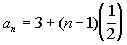.

 Since an arithmetic sequence is a sequence, you find the terms exactly the same way that you do a sequence.   n is our term number and we plug the term number into the function to find the value of the term. If you need a review on sequences, feel free to go to Tutorial 54A: Sequences. Lets see what we get for our first five terms: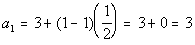*n = 1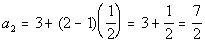*n = 2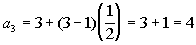*n = 3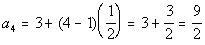*n = 4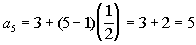*n = 5

 Note how the first term is 3. What was the common difference for this arithmetic sequence? If you said ½, you are correct!  Note how each term went up by 1/2 from the previous term. Now lets check out the 15th term: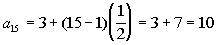*n = 15Example 2:   Find the first five terms and the15th term of the arithmetic sequence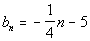.

 Since an arithmetic sequence is a sequence, you find the terms exactly the same way that you do a sequence.   n is our term number and we plug the term number into the function to find the value of the term. If you need a review on sequences, feel free to go to Tutorial 54A: Sequences. Lets see what we get for our first five terms: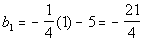*n = 1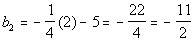*n = 2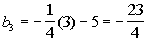*n = 3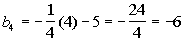*n = 4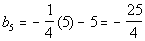*n = 5

 Note how the first term is not -5, but -21/4.  The formula for the nth term used n, instead of n - 1 that is in the general form.  So it was a simplified or adjusted form that was used. What was the common difference for this arithmetic sequence? If you said -1/4 you are correct!  Note how each term went DOWN by 1/4 from the previous term. Now lets check out the 15th term: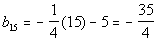*n = 15Example 3:   Write a formula for the nth term of the arithmetic sequence -10, -5, 0, 5, ....

 We will use the nth term formula for an arithmetic sequence,, to help us with this problem. Basically we need to find two things, the first term of the sequenceand the common difference, d. What is, the first term of the sequence? If you said -10, give yourself a high five.  The first term of this sequence is -10. What is d, the common difference? If you said 5, you are right!!  Note that you would have to add 5 to each previous term to get to the next term: -10 + 5 = -5, -5 + 5 = 0, and 0 + 5 = 5.   It has to be consistent throughout the sequence. Putting in -10 forand 5 for d we get:Either of these answers would give you the same arithmetic sequence.Example 4:   Write a formula for the nth term of the arithmetic sequence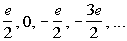We will use the nth term formula for an arithmetic sequence,, to help us with this problem. Basically we need to find two things, the first term of the sequenceand the common difference, d. What is, the first term of the sequence? If you said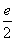, give yourself a high five.  The first term of this sequence is. What is d, the common difference? If you said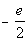, you are right!!  Note that you would have to addto each previous term to get to the next term. Putting inforandfor d we get: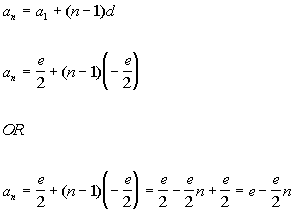Either of these answers would give you the same arithmetic sequence.

 Arithmetic Series The Sum of the First n Terms  of an Arithmetic Sequenceis the first term of the sequence and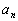is the nth term of the sequence.

 If you need a review on sequences, feel free to go to Tutorial 54B: Series.Example 5:   Find the sum of the arithmetic series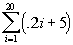.

 Note how i starts at 1 and ends at 20.  So we are looking for the sum of terms 1 - 20.   We will use the formula for the sum of the first n terms of an arithmetic sequence,, to help us with this problem. Basically we need to find three things: the first term of the sequence, the last term of the sequence, and how many terms of the sequence we are adding in the series. What is the first term? If you said 5.2 you are right!  You get that by plugging 1 into the given formula: .2i + 5 .2(1) + 5 = 5.2 What is the last term? If you said 9, give yourself a pat on the back.  You get that by plugging 20 into the given formula: .2i + 5 .2(20) + 5 = 9 How many terms are we summing up? If you said 20, you are correct.  If you start at 1 and go all the way to 20, there will be 20 terms. Putting in 5.2 for the first term, 9 for the last term, and 20 for n, we get: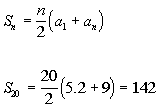Example 6:   Find the sum of the arithmetic series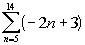.

 Note how n starts at 5 and ends at 14.  So we are looking for the sum of terms 5 - 14. We will use the formula for the sum of the first n terms of an arithmetic sequence,, to help us with this problem. Basically we need to find three things: the first term of the sequence, the last term of the sequence, and how many terms of the sequence we are adding in the series. What is the first term? If you said -7 you are right!  Since this summation starts at 5, you need to plug in 5 into the given formula: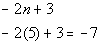What is the last term? If you said -25 give yourself a pat on the back. You get that by plugging 14 into the given formula: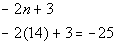How many terms are we summing up? If you said 10, you are correct.  If you start at 5 and go all the way to 14, there will be 10 terms. Putting in -7 for the first term, -25 for the last term, and 10 for n, we get: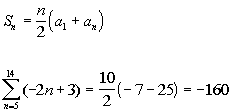Example 7:   Find the sum of the arithmetic series 3 + 6 + 9 + .... + 99.

 We will use the formula for the sum of the first n terms of an arithmetic sequence,, to help us with this problem. Basically we need to find three things: the first term of the sequence, the last term of the sequence, and how many terms of the sequence we are adding in the series. What is the first term? If you said 3 you are right!  What is the last term? If you said 99 give yourself a pat on the back. How many terms are we summing up? If you said 33, you are correct. Because of the way it is written with the 3 dots, this one is a little bit trickier.  You have to do a little figuring.  We can use the nth term of an arithmetic sequence and solve for n, the number of terms in the sequence: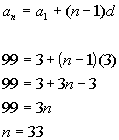Putting in 3 for the first term, 99 for the last term, and 33 for n, we get: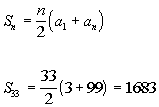Practice Problems

 These are practice problems to help bring you to the next level.  It will allow you to check and see if you have an understanding of these types of problems. Math works just like anything else, if you want to get good at it, then you need to practice it.  Even the best athletes and musicians had help along the way and lots of practice, practice, practice, to get good at their sport or instrument.  In fact there is no such thing as too much practice. To get the most out of these, you should work the problem out on your own and then check your answer by clicking on the link for the answer/discussion for that  problem.  At the link you will find the answer as well as any steps that went into finding that answer.Practice Problems 1a - 1b: Find the first five terms and the 15th term of the given arithmetic sequence.

 1a.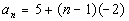(answer/discussion to 1a) 1b.(answer/discussion to 1b)Practice Problems 2a - 2b:Write a formula for the nth term of the given arithmetic sequence.

 2a. 0, .25, .5, .75, ... (answer/discussion to 2a) 2b. -5, -15, -25, -35, ... (answer/discussion to 2b)Practice Problems 3a - 3b: Find the given term of the expansion.  Simplify the results.

 3a. 5 + 10 + 15 +.... + 500 (answer/discussion to 3a) 3b.(answer/discussion to 3b)Need Extra Help on these Topics?

 There were no good websites found to help us with the topics on this page.   Go to Get Help Outside the Classroom found in Tutorial 1: How to Succeed in a Math Class for some more suggestions.

Last revised on May 17, 2011 by Kim Seward.